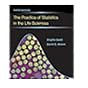Normal view MARC view ISBD view

# Mathematics for the life sciences : calculus, modeling, probability, and dynamical systems / Glenn Ledder.

Material type:BookCopyright date: New York, Springer, 2013Edition: First Edition.Description: xx, 431 pages : illustrations ; 27 cm.ISBN: 9781461472759.DDC classification: 570.15118
Contents:
A brief summary of calculus -- Mathematical modeling -- Probability distributions -- Working with probability -- Dynamics of single populations -- Discrete dynamical systems -- Continuous dynamical systems -- Appendix A. Additional topics in discrete dynamical systems -- Appendix B. The definite integral via Riemann sums -- Appendix C. A Runge-Kutta method for numerical solution of differential equations -- Hints and answeres to selected problems.
Summary: Mathematics for the Life Sciences provides present and future biologists with the mathematical concepts and tools needed to understand and use mathematical models and read advanced mathematical biology books. It presents mathematics in biological contexts, focusing on the central mathematical ideas, and providing detailed explanations. The author assumes no mathematics background beyond algebra and precalculus. Calculus is presented as a one-chapter primer that is suitable for readers who have not studied the subject before, as well as readers who have taken a calculus course and need a review. This primer is followed by a novel chapter on mathematical modeling that begins with discussions of biological data and the basic principles of modeling. The remainder of the chapter introduces the reader to topics in mechanistic modeling (deriving models from biological assumptions) and empirical modeling (using data to parameterize and select models). The modeling chapter contains a thorough treatment of key ideas and techniques that are often neglected in mathematics books. It also provides the reader with a sophisticated viewpoint and the essential background needed to make full use of the remainder of the book, which includes two chapters on probability and its applications to inferential statistics and three chapters on discrete and continuous dynamical systems. The biological content of the book is self-contained and includes many basic biology topics such as the genetic code, Mendelian genetics, population dynamics, predator-prey relationships, epidemiology, and immunology. The large number of problem sets include some drill problems along with a large number of case studies. The latter are divided into step-by-step problems and sorted into the appropriate section, allowing readers to gradually develop complete investigations from understanding the biological assumptions to a complete analysis.
Tags from this library: No tags from this library for this title.
Item type Current location Call number Copy number Status Date due Item holdsLibro académico
570.15118 L472m 2013 (Browse shelf) Ej. 1 AvailableLibro académico
570.15118 L472m 2013 (Browse shelf) Ej. 2 AvailableLibro académico
570.15118 L472m 2013 (Browse shelf) Ej. 3 Available
Total holds: 0
##### Browsing Biblioteca del Campus Shelves Close shelf browser570.151 H128m 1982 Matemáticas para biólogos / 570.15118 L472m 2013 Mathematics for the life sciences : 570.15118 L472m 2013 Mathematics for the life sciences : 570.15118 L472m 2013 Mathematics for the life sciences : 570.1515 S3785c 2014 Calculus for the life sciences / 570.15195 B177p 2014 The practice of statistics in the life sciences / 570.15195 B177p 2014 The practice of statistics in the life sciences /

Includes bibliographical references and index.

A brief summary of calculus -- Mathematical modeling -- Probability distributions -- Working with probability -- Dynamics of single populations -- Discrete dynamical systems -- Continuous dynamical systems -- Appendix A. Additional topics in discrete dynamical systems -- Appendix B. The definite integral via Riemann sums -- Appendix C. A Runge-Kutta method for numerical solution of differential equations -- Hints and answeres to selected problems.

Mathematics for the Life Sciences provides present and future biologists with the mathematical concepts and tools needed to understand and use mathematical models and read advanced mathematical biology books. It presents mathematics in biological contexts, focusing on the central mathematical ideas, and providing detailed explanations. The author assumes no mathematics background beyond algebra and precalculus. Calculus is presented as a one-chapter primer that is suitable for readers who have not studied the subject before, as well as readers who have taken a calculus course and need a review. This primer is followed by a novel chapter on mathematical modeling that begins with discussions of biological data and the basic principles of modeling. The remainder of the chapter introduces the reader to topics in mechanistic modeling (deriving models from biological assumptions) and empirical modeling (using data to parameterize and select models). The modeling chapter contains a thorough treatment of key ideas and techniques that are often neglected in mathematics books. It also provides the reader with a sophisticated viewpoint and the essential background needed to make full use of the remainder of the book, which includes two chapters on probability and its applications to inferential statistics and three chapters on discrete and continuous dynamical systems. The biological content of the book is self-contained and includes many basic biology topics such as the genetic code, Mendelian genetics, population dynamics, predator-prey relationships, epidemiology, and immunology. The large number of problem sets include some drill problems along with a large number of case studies. The latter are divided into step-by-step problems and sorted into the appropriate section, allowing readers to gradually develop complete investigations from understanding the biological assumptions to a complete analysis.

There are no comments for this item.